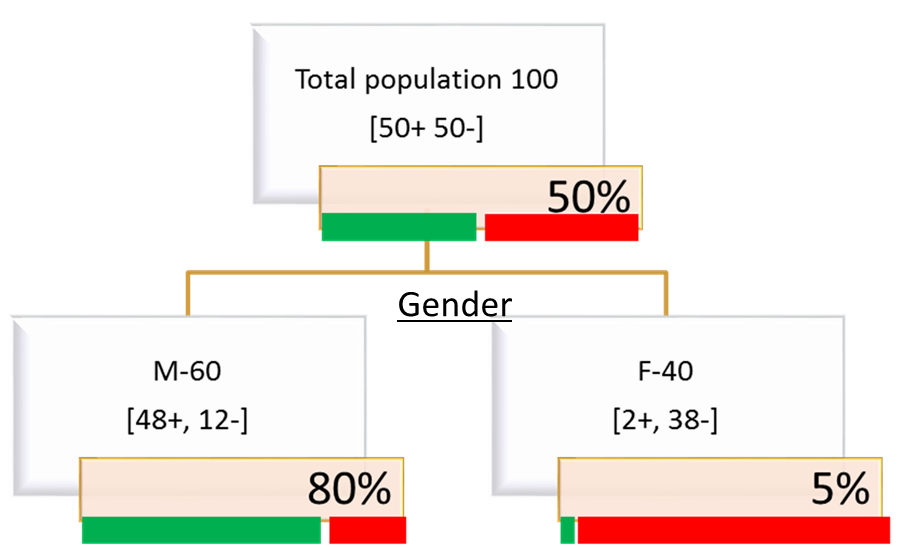• No products in the cart.

# 203.3.4 How to Calculate Entropy for Decision Tree Split?

### LAB: Entropy Calculation – Example

In previous section, we studied about  How Decision tree Splits works?

• Calculate entropy at the root for the given population
• Calculate the entropy for the two distinct gender segments### Code- Entropy Calculation

• Entropy at root 100%
• Male Segment : (-48/60)log(48/60,2)-(12/60)log(12/60,2)
• 0.7219281
• FemaleSegment : (-2/40)log(2/40,2)-(38/40)log(38/40,2)
• 0.286397The next post is about Information Gain in Decision tree Split.

20th June 2017
0 responses on "203.3.4 How to Calculate Entropy for Decision Tree Split?"